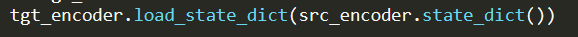# What do these methods in pytorch do?

I have read a lot of explanations for the following methods, but I still don’t understand the specific use. Please explain, thank you!2. .data , .max(1)3. .detach()1. Load the `state_dict`, which contains all parameters and buffers of this module, into `tgt_encoder`, which seems to be a model (based on the name).

2. `preds.max(1)` calculates the max values and indices in dim1 and returns the indices (by indexing the result as ``). Don’t use the `.data` attribute, as it might have unwanted side effects. Alternatively you could also use `torch.argmax(preds, 1)` to get the indices. This is often used to get the prediction of a model output, which contains logits or log_probabilities.

3. `detach()` detaches the tensor from the computation graph. Autograd will stop at this point and will not calculate any gradients for the previous operations involving `feat_concat`.

1. Does this mean that the parameters in the two models src_encoder and tgt_encoder are exactly the same? What are buffers?

2. I still can’t understand the meaning of .max (1) . This is context. Can you tell me what it does? Or tell me how should I modify the code?3. Can you explain what the computation graph is?

1. Yes, at this point both models contains the same parameters and buffers. If they are trained afterwards, the values might of course diverge. Buffers are non-trainable tensors, which are registered to the module, e.g. the running stats of batchnorm layers.

2. Assuming `preds` have the shape `[batch_size, nb_classes, *]` and return the logits/log_prbabilities, you’ll get the predicted classes using this code (same output with the `.max(1)` call):

``````batch_size, nb_classes = 2, 10
output = torch.randn(batch_size, nb_classes)
print(output)
> tensor([[-2.1836, -0.8442,  0.7195, -0.6133,  0.8548,  0.5433,  0.1492, -0.3216,
0.1395, -0.5018],
[-1.3000,  0.1784, -0.1760, -0.1664, -0.5358, -0.2816, -1.4170,  0.4686,
0.8357, -0.0534]])
pred = torch.argmax(output, 1)
print(pred)
> tensor([4, 8])
``````
1. PyTorch creates a computation graph dynamically in the forward pass, which is then used in the backward pass to backpropagate and compute the gradients.

Does this line of code in question 2 mean that the index of pred_cls and labels is the same? Is it right to use the category index for comparison?

``acc += pred_cls.eq(labels.data).cpu().sum()``

If the predicted indices and target indices are equal, your model predicted the right classes, otherwise the wrong ones.

Your code will give you the sum of correctly predicted classes, which is not the accuracy.
However, if you divide by the number of samples afterwards, it should work.

Also, don’t use the `.data` attribute as mentioned before.1 Like

OK, thank you very much for answering all my questions.